Q-Chem 5.0 User’s Manual

# 5.10 Coupled Cluster Active Space Methods

## 5.10.1 Introduction

Electron correlation effects can be qualitatively divided into two classes. The first class is static or non-dynamical correlation: long wavelength low-energy correlations associated with other electron configurations that are nearly as low in energy as the lowest energy configuration. These correlation effects are important for problems such as homolytic bond breaking, and are the hardest to describe because by definition the single configuration Hartree-Fock description is not a good starting point. The second class is dynamical correlation: short wavelength high-energy correlations associated with atomic-like effects. Dynamical correlation is essential for quantitative  accuracy, but a reasonable description of static correlation is a prerequisite for a calculation being qualitatively  correct.

In the methods discussed in the previous several subsections, the objective was to approximate the total correlation energy. However, in some cases, it is useful to model directly the non-dynamical and dynamical correlation energies separately. The reasons for this are pragmatic: with approximate methods, such a separation can give a more balanced treatment of electron correlation along bond-breaking coordinates, or reaction coordinates that involve diradicaloid intermediates. The non-dynamical correlation energy is conveniently defined as the solution of the Schrödinger equation within a small basis set composed of valence bonding, anti-bonding and lone pair orbitals: the so-called full valence active space. Solved exactly, this is the so-called full valence complete active space SCF (CASSCF) , or equivalently, the fully optimized reaction space (FORS) method .

Full valence CASSCF and FORS involve computational complexity which increases exponentially with the number of atoms, and is thus unfeasible beyond systems of only a few atoms, unless the active space is further restricted on a case-by-case basis. Q-Chem includes two relatively economical methods that directly approximate these theories using a truncated coupled-cluster doubles wave function with optimized orbitals . They are active space generalizations of the OD and QCCD methods discussed previously in Sections 5.8.3 and 5.8.4, and are discussed in the following two subsections. By contrast with the exponential growth of computational cost with problem size associated with exact solution of the full valence CASSCF problem, these cluster approximations have only 6th-order growth of computational cost with problem size, while often providing useful accuracy.

The full valence space is a well-defined theoretical chemical model. For these active space coupled-cluster doubles methods, it consists of the union of valence  levels that are occupied in the single determinant reference, and those that are empty. The occupied levels that are to be replaced can only be the occupied valence and lone pair orbitals, whose number is defined by the sum of the valence electron counts for each atom (i.e., 1 for H, 2 for He, 1 for Li, etc..). At the same time, the empty virtual orbitals to which the double substitutions occur are restricted to be empty (usually anti-bonding) valence orbitals. Their number is the difference between the number of valence atomic orbitals, and the number of occupied valence orbitals given above. This definition (the full valence space) is the default when either of the “valence” active space methods are invoked (VOD or VQCCD)

There is also a second useful definition of a valence active space, which we shall call the 1:1 or perfect pairing active space. In this definition, the number of occupied valence orbitals remains the same as above. The number of empty correlating orbitals in the active space is defined as being exactly the same number, so that each occupied orbital may be regarded as being associated 1:1 with a correlating virtual orbital. In the water molecule, for example, this means that the lone pair electrons as well as the bond-orbitals are correlated. Generally the 1:1 active space recovers more correlation for molecules dominated by elements on the right of the periodic table, while the full valence active space recovers more correlation for molecules dominated by atoms to the left of the periodic table.

If you wish to specify either the 1:1 active space as described above, or some other choice of active space based on your particular chemical problem, then you must specify the numbers of active occupied and virtual orbitals. This is done via the standard “window options”, documented earlier in this Chapter.

Finally we note that the entire discussion of active spaces here leads only to specific numbers of active occupied and virtual orbitals. The orbitals that are contained within these spaces are optimized by minimizing the trial energy with respect to all the degrees of freedom previously discussed: the substitution amplitudes, and the orbital rotation angles mixing occupied and virtual levels. In addition, there are new orbital degrees of freedom to be optimized to obtain the best active space of the chosen size, in the sense of yielding the lowest coupled-cluster energy. Thus rotation angles mixing active and inactive occupied orbitals must be varied until the energy is stationary. Denoting inactive orbitals by primes and active orbitals without primes, this corresponds to satisfying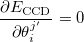(5.39)

Likewise, the rotation angles mixing active and inactive virtual orbitals must also be varied until the coupled-cluster energy is minimized with respect to these degrees of freedom: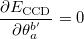(5.40)

## 5.10.2 VOD and VOD(2) Methods

The VOD method is the active space version of the OD method described earlier in Section 5.8.3. Both energies and gradients are available for VOD, so structure optimization is possible. There are a few important comments to make about the usefulness of VOD. First, it is a method that is capable of accurately treating problems that fundamentally involve 2 active electrons in a given local region of the molecule. It is therefore a good alternative for describing single bond-breaking, or torsion around a double bond, or some classes of diradicals. However it often performs poorly for problems where there is more than one bond being broken in a local region, with the non variational solutions being quite possible. For such problems the newer VQCCD method is substantially more reliable.

Assuming that VOD is a valid zero order description for the electronic structure, then a second-order correction, VOD(2), is available for energies only. VOD(2) is a version of OD(2) generalized to valence active spaces. It permits more accurate calculations of relative energies by accounting for dynamical correlation.

## 5.10.3 VQCCD

The VQCCD method is the active space version of the QCCD method described earlier in Section 5.8.3. Both energies and gradients are available for VQCCD, so that structure optimization is possible. VQCCD is applicable to a substantially wider range of problems than the VOD method, because the modified energy functional is not vulnerable to non variational collapse. Testing to date suggests that it is capable of describing double bond breaking to similar accuracy as full valence CASSCF, and that potential curves for triple bond-breaking are qualitatively correct, although quantitatively in error by a few tens of kcal/mol. The computational cost scales in the same manner with system size as the VOD method, albeit with a significantly larger prefactor.

## 5.10.4 Local Pair Models for Valence Correlations Beyond Doubles

Working with Prof. Head-Gordon at Berkeley, John Parkhill has developed implementations for pair models which couple 4 and 6 electrons together quantitatively. Because these truncate the coupled cluster equations at quadruples and hextuples respectively they have been termed the “Perfect Quadruples” and “Perfect Hextuples” models. These can be viewed as local approximations to CASSCF. The PQ and PH models are executed through an extension of Q-Chem’s coupled cluster code, and several options defined for those models will have the same effects although the mechanism may be different (CC_DIIS_START, CC_DIIS_SIZE, CC_DOV_THRESH, CC_CONV, etc.).

In the course of implementation, the non-local coupled cluster models were also implemented up to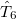. Because the algorithms are explicitly sparse their costs relative to the existing implementations of CCSD are much higher (and should never be used in lieu of an existing CCMAN code), but this capability may be useful for development purposes, and when computable, models above CCSDTQ are highly accurate. To use PQ, PH, their dynamically correlated “+SD” versions or this machine generated cluster code set: METHOD = MGC.

MGC_AMODEL
 Choice of approximate cluster model.

TYPE:
 INTEGER

DEFAULT:
 Determines how the CC equations are approximated:

OPTIONS:
 0 Local Active-Space Amplitude iterations (pre-calculate GVB orbitals with your method of choice (RPP is good)). 7 Optimize-Orbitals using the VOD 2-step solver. (Experimental-only use with MGC_AMPS = 2, 24 ,246) 8 Traditional Coupled Cluster up to CCSDTQPH. 9 MR-CC version of the Pair-Models. (Experimental)

RECOMMENDATION:
 None

MGC_NLPAIRS
 Number of local pairs on an amplitude.

TYPE:
 INTEGER

DEFAULT:
 None

OPTIONS:
 Must be greater than 1, which corresponds to the PP model. 2 for PQ, and 3 for PH.

RECOMMENDATION:
 None

MGC_AMPS
 Choice of Amplitude Truncation

TYPE:
 INTEGER

DEFAULT:
 None

OPTIONS:
 2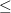n123456, a sorted list of integers for every amplitude which will be iterated. Choose 1234 for PQ and 123456 for PH

RECOMMENDATION:
 None

MGC_LOCALINTS
 Pair filter on an integrals.

TYPE:
 LOGICAL

DEFAULT:
 FALSE

OPTIONS:
 Enforces a pair filter on the 2-electron integrals, significantly reducing computational cost. Generally useful. for more than 1 pair locality.

RECOMMENDATION:
 None

MGC_LOCALINTER
 Pair filter on an intermediate.

TYPE:
 LOGICAL

DEFAULT:
 FALSE

OPTIONS:
 Any nonzero value enforces the pair constraint on intermediates, significantly reducing computational cost. Not recommended for2 pair locality

RECOMMENDATION:
 None

## 5.10.5 Convergence Strategies and More Advanced Options

These optimized orbital coupled-cluster active space methods enable the use of the full valence space for larger systems than is possible with conventional complete active space codes. However, we should note at the outset that often there are substantial challenges in converging valence active space calculations (and even sometimes optimized orbital coupled cluster calculations without an active space). Active space calculations cannot be regarded as “routine” calculations in the same way as SCF calculations, and often require a considerable amount of computational trial and error to persuade them to converge. These difficulties are largely because of strong coupling between the orbital degrees of freedom and the amplitude degrees of freedom, as well as the fact that the energy surface is often quite flat with respect to the orbital variations defining the active space.

Being aware of this at the outset, and realizing that the program has nothing against you personally is useful information for the uninitiated user of these methods. What the program does have, to assist in the struggle to achieve a converged solution, are accordingly many convergence options, fully documented in Appendix C. In this section, we describe the basic options and the ideas behind using them as a starting point. Experience plays a critical role, however, and so we encourage you to experiment with toy jobs that give rapid feedback in order to become proficient at diagnosing problems.

If the default procedure fails to converge, the first useful option to employ is CC_PRECONV_T2Z, with a value of between 10 and 50. This is useful for jobs in which the MP2 amplitudes are very poor guesses for the converged cluster amplitudes, and therefore initial iterations varying only the amplitudes will be beneficial:

CC_PRECONV_T2Z
 Whether to pre-converge the cluster amplitudes before beginning orbital optimization in optimized orbital cluster methods.

TYPE:
 INTEGER

DEFAULT:
 0 (FALSE) 10 If CC_RESTART, CC_RESTART_NO_SCF or CC_MP2NO_GUESS are TRUE

OPTIONS:
 0 No pre-convergence before orbital optimization.Up toiterations in this pre-convergence procedure.

RECOMMENDATION:
 Experiment with this option in cases of convergence failure.

Other options that are useful include those that permit some damping of step sizes, and modify or disable the standard DIIS procedure. The main choices are as follows.

CC_DIIS
 Specify the version of Pulay’s Direct Inversion of the Iterative Subspace (DIIS) convergence accelerator to be used in the coupled-cluster code.

TYPE:
 INTEGER

DEFAULT:
 0

OPTIONS:
 0 Activates procedure 2 initially, and procedure 1 when gradients are smaller than DIIS12_SWITCH. 1 Uses error vectors defined as differences between parameter vectors from successive iterations. Most efficient near convergence. 2 Error vectors are defined as gradients scaled by square root of the approximate diagonal Hessian. Most efficient far from convergence.

RECOMMENDATION:
 DIIS1 can be more stable. If DIIS problems are encountered in the early stages of a calculation (when gradients are large) try DIIS1.

CC_DIIS_START
 Iteration number when DIIS is turned on. Set to a large number to disable DIIS.

TYPE:
 INTEGER

DEFAULT:
 3

OPTIONS:User-defined

RECOMMENDATION:
 Occasionally DIIS can cause optimized orbital coupled-cluster calculations to diverge through large orbital changes. If this is seen, DIIS should be disabled.

CC_DOV_THRESH
 Specifies minimum allowed values for the coupled-cluster energy denominators. Smaller values are replaced by this constant during early iterations only, so the final results are unaffected, but initial convergence is improved when the guess is poor.

TYPE:
 INTEGER

DEFAULT: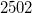Corresponding to 0.25

OPTIONS: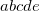Integer code is mapped to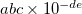RECOMMENDATION:
 Increase to 0.5 or 0.75 for non convergent coupled-cluster calculations.

CC_THETA_STEPSIZE
 Scale factor for the orbital rotation step size. The optimal rotation steps should be approximately equal to the gradient vector.

TYPE:
 INTEGER

DEFAULT: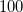Corresponding to 1.0

OPTIONS:Integer code is mapped toIf the initial step is smaller than 0.5, the program will increase step when gradients are smaller than the value of THETA_GRAD_THRESH, up to a limit of 0.5.

RECOMMENDATION:
 Try a smaller value in cases of poor convergence and very large orbital gradients. For example, a value of 01001 translates to 0.1

An even stronger—and more-or-less last resort—option permits iteration of the cluster amplitudes without changing the orbitals:

CC_PRECONV_T2Z_EACH
 Whether to pre-converge the cluster amplitudes before each change of the orbitals in optimized orbital coupled-cluster methods. The maximum number of iterations in this pre-convergence procedure is given by the value of this parameter.

TYPE:
 INTEGER

DEFAULT:
 0 (FALSE)

OPTIONS:
 0 No pre-convergence before orbital optimization.Up toiterations in this pre-convergence procedure.

RECOMMENDATION:
 A very slow last resort option for jobs that do not converge.

## 5.10.6 Examples

Example 5.94  Two jobs that compare the correlation energy of the water molecule with partially stretched bonds, calculated via the two coupled-cluster active space methods, VOD, and VQCCD. These are relatively “easy” jobs to converge, and may be contrasted with the next example, which is not easy to converge. The orbitals are restricted.

$molecule 0 1 O H 1 r H 1 r a r = 1.5 a = 104.5$end

$rem METHOD vod BASIS 6-31G$end

@@@

$molecule read$end

$rem METHOD vqccd BASIS 6-31G$end


Example 5.95  The water molecule with highly stretched bonds, calculated via the two coupled-cluster active space methods, VOD, and VQCCD. These are “difficult” jobs to converge. The convergence options shown permitted the job to converge after some experimentation (thanks due to Ed Byrd for this!). The difficulty of converging this job should be contrasted with the previous example where the bonds were less stretched. In this case, the VQCCD method yields far better results than VOD!.

$molecule 0 1 O H 1 r H 1 r a r = 3.0 a = 104.5$end

$rem METHOD vod BASIS 6-31G SCF_CONVERGENCE 9 THRESH 12 CC_PRECONV_T2Z 50 CC_PRECONV_T2Z_EACH 50 CC_DOV_THRESH 7500 CC_THETA_STEPSIZE 3200 CC_DIIS_START 75$end

@@@

$molecule read$end

$rem METHOD vqccd BASIS 6-31G SCF_CONVERGENCE 9 THRESH 12 CC_PRECONV_T2Z 50 CC_PRECONV_T2Z_EACH 50 CC_DOV_THRESH 7500 CC_THETA_STEPSIZE 3200 CC_DIIS_START 75$end